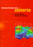© CAMBRIDGE UNIVERSITY PRESS 1999

1.6. Origin of Fluctuations: Inflation and Topological Defects

1.6.1 Topological defects

A fundamental scalar field, the Higgs field, is invoked by particle theorists to account for the generation of mass; one of the main goals of the next generation of particle accelerators, including the Large Hadron Collider at CERN, will be to verify the Higgs theory for the generation of the mass of the weak vector bosons and all the lighter elementary particles. Another scalar field is required to produce the vacuum energy which may drive cosmic inflation (discussed in the next section). Scalar fields can also create topological defects that might be of great importance in cosmology. The basic idea is that some symmetry is broken wherever a given scalar field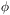has a non-vanishing value, so the dimensionality of the corresponding topological defect depends on the number of components of the scalar field: for a single-component real scalar field,(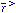) = 0 defines a two-dimensional surface in three-dimensional space, a domain wall; for a complex scalar field, the real and imaginary parts of() = 0 define a one-dimensional locus, a cosmic string; for a three-component (e.g., isovector) field,i() = 0 for i = 1, 2, 3 is satisfied at isolated points, monopoles; for more than three components, one gets textures that are not topologically stable but which can seed structure in the universe as they unwind.

To see how this works in more detail, consider a cosmic string. For the underlying field theory to permit cosmic strings, we need to couple a complex scalar fieldto a single-component (i.e., U(1)) gauge field A, like the electromagnetic field, in the usual way via the substitution ð-> D- ie A), so that the scalar field derivative term in the Lagrangian becomesD= |D|2. Then if the scalar fieldgets a non-zero value by the usual Higgs ``spontaneous symmetry breaking'' mechanism, the gauge symmetry is broken because the field has a definite complex phase. But along a string where= 0 the symmetry is restored. As one circles around the string at any point on it, the complex phase of() in general makes one, or possibly n > 1, complete circles 0 -> 2 n. But since such a phase rotation can be removed at large distance from the string by a gauge transformation ofand A, the energy density associated with this behavior ofD-> 0 at large distances, and therefore the energy µ per unit length of string is finite. Since it would require an infinite amount of energy to unwind the phase ofat infinity, however, the string is topologically stable. If the field theory describing the early universe includes a U(1) gauge field and associated complex Higgs field, a rather high density of such cosmic strings will form when the string fieldacquires its nonzero value and breaks the U(1) symmetry. This happens because there is no way for the phase ofto be aligned in causally disconnected regions, and it is geometrically fairly likely that the phases will actually wrap around as required for a string to go through a given region (Kibble 1976). The string network will then evolve and can help cause formation of structure after the universe becomes matter dominated, as long as the string density is not diluted by a subsequent period of cosmic inflation (on the difficult problem of combining cosmic defects and inflation, see, e.g., Hodges & Primack 1991). A similar discussion can be given for domain walls and local (gauge) monopoles, but these objects are cosmologically pathological since they dominate the energy density and ``overclose'' the universe. But cosmic strings, a sufficiently low density of global (i.e., non-gauged monopoles), and global textures are potentially interesting for cosmology (recent reviews include Vilenkin & Shellard 1994, Hindmarsh & Kibble 1995, Shellard 1996). Cosmic defects are the most important class of models producing non-Gaussian fluctuations which could seed cosmic structure formation. Since they are geometrically extended objects, they correspond to non-local non-Gaussian fluctuations (Kofman et al. 1991).

The parameter µ, usually quoted in the dimensionless form (where G is Newton's constant), is the key parameter of the theory of cosmic strings. The value required for the COBE normalization is 6x 106 = 1-2 (recent determinations include 6 = 1.7 ± 0.7, Perivolaropoulos 1993; 2, Coulson et al. 1994; (1.05-0.20+0.35), Allen et al. 1996; 1.7, Allen et al. 1997). This is close enough to the value required for structure formation, = (2.2-2.8) b8-1 x 10-6 (Albrecht & Stebbins 1992), with the smaller value for cosmic strings plus cold dark matter and the higher value for cosmic strings plus hot dark matter, so that the necessary value of the biasing factor b8 is 1.3-3, which is high (probably leading to underproduction of clusters, and large-scale velocities that are low compared to observations - cf. Perivolaropoulos & Vachaspati 1994), but perhaps not completely crazy. (Here b8 is the factor by which galaxies must be more clustered than dark matter, on a scale of 8 h-1 Mpc.) Since generically ~ (M / mpl)2, where M is the energy scale at which the string fieldacquires its nonzero value, the fact that ~ 10-6, corresponding to M at roughly the Grand Unification scale, is usually regarded as a plus for the cosmic string scenario. (Even though there is no particular necessity for cosmic strings in GUT scenarios, GUT groups larger than the minimal SU(5) typically do contain the needed extra U(1)s.) Moreover, the required normalization is well below the upper limit obtained from the requirement that the gravitational radiation generated by the evolution of the string network not disrupt Big Bang Nucleosynthesis,6 x 10-6. However, there is currently controversy whether it is also below the upper limit from pulsar timing, which has been determined to be6 x 10-7 (Thorsett & Dewey 1996) vs.5 x 10-6 (McHugh et al. 1996; cf. Caldwell, Battye, & Shellard 1996).

As for cosmic strings, the COBE normalization for global texture models also implies a high bias b83.4 for h = 0.7 (Bennett & Rhie 1993), although the needed bias is somewhat lower for0.3 (Pen & Spergel 1995). The latest global defect simulations (Pen, Seljak, & Turok, 1997) show that the matter power spectrum in all such models also has a shape very different than that suggested by the available data on galaxies and clusters.

But both cosmic string and global defect models have a problem which may be even more serious: they predict a small-angle CMB fluctuation spectrum in which the first peak is at rather high angular wavenumber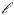~ 400 (Crittenden & Turok 1995, Durrer et al. 1996, Magueijo et al. 1996) and in any case is rather low in amplitude, partly because of incoherent addition of scalar, vector, and tensor modes, according to the latest simulations (strings: Allen et al. 1997; global defects: Pen, Seljak, & Turok 1997; cf. Albrecht, Battye, & Robinson 1997). This is in conflict with the currently available small-angle CMB data (Netterfield et al. 1997, Scott et al. 1996), which shows a peak at~ 250 and a drop at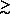400, as predicted by flat (0 += 1) CDM-type models. Since the small-angle CMB data is still rather preliminary, it is premature to regard the cosmic defect models as being definitively ruled out. It will be interesting to see the nature of the predicted galaxy distribution and CMB anisotropies when more complete simulations of cosmic defect models are run. This is more difficult than simulating models with the usual inflationary fluctuations, both because it is necessary to evolve the defects, and also because the fact that these defects represent rare but high amplitude fluctuations necessitates a careful treatment of their local effects on the ordinary and dark matter. It may be difficult to sustain the effort such calculations require, because the poor agreement between the latest defect simulations and current small-angle CMB data does not bode well for defect theories. Fortunately, there have been significant technical breakthroughs in calculational techniques (cf. Allen et al. 1997, Pen et al. 1997).